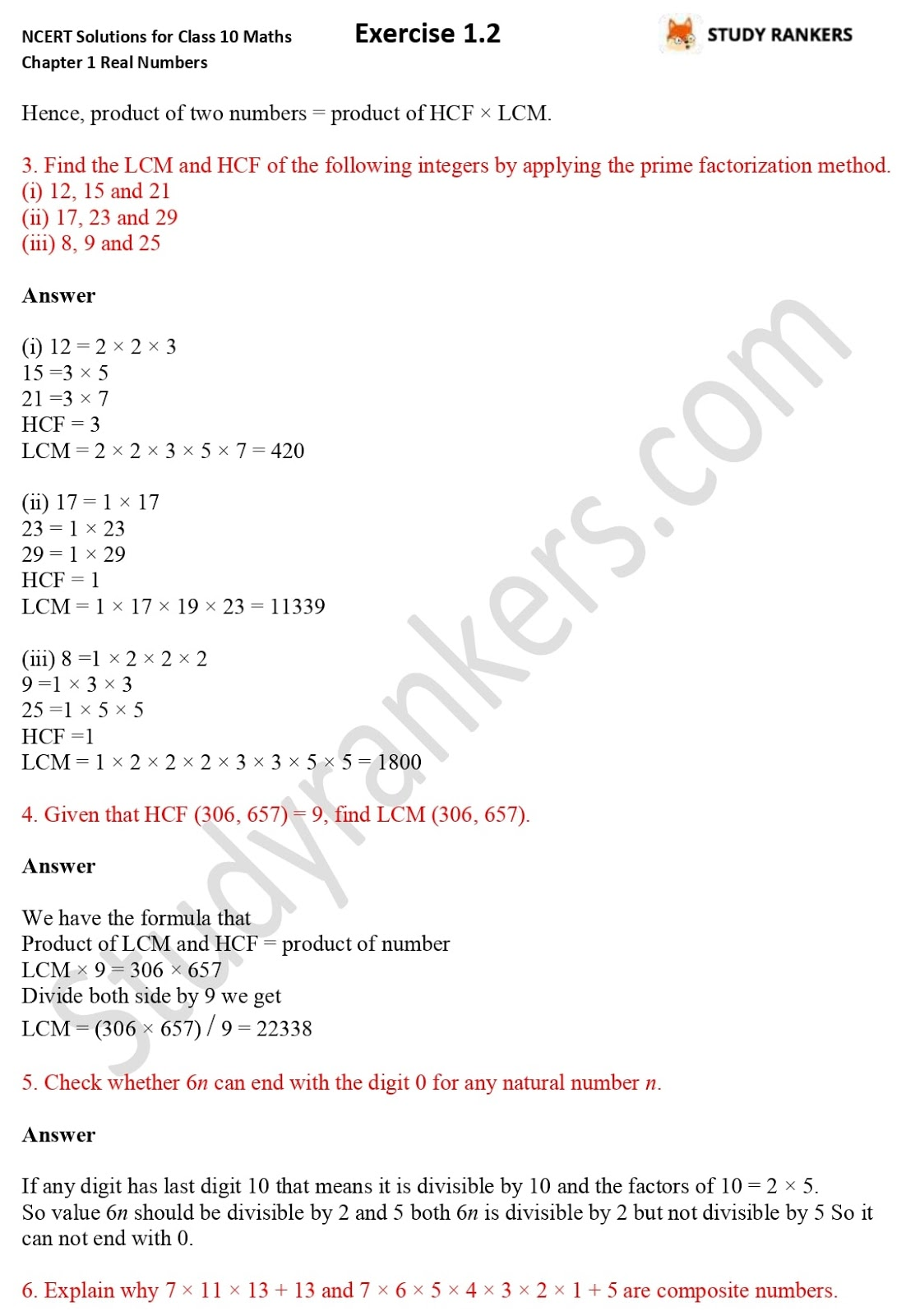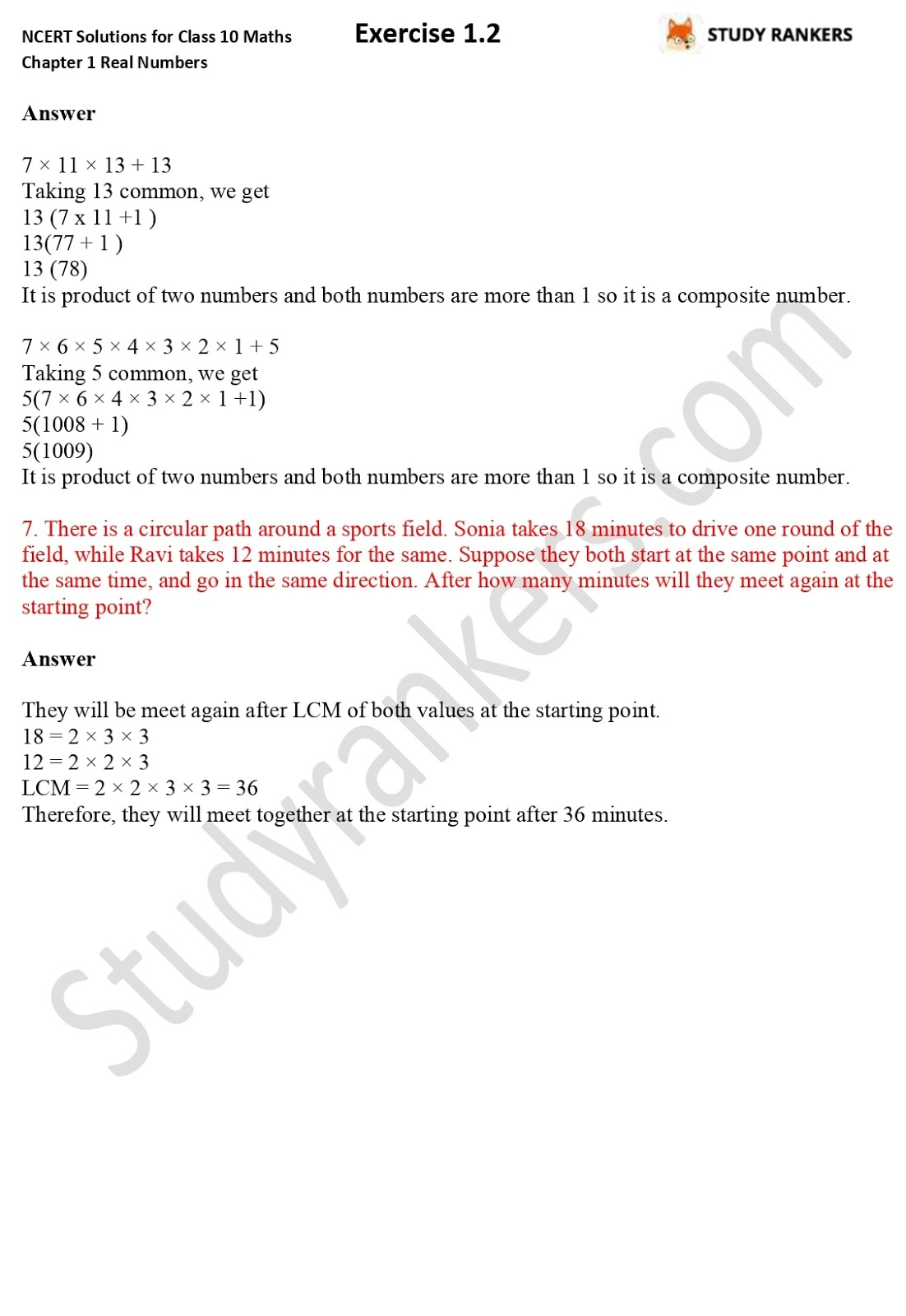## NCERT Solutions for Class 10 Maths Chapter 1 Real Numbers Exercise 1.2

Chapter 1 Real Numbers Exercise 1.2 NCERT Solutions for Class 10 Maths is given here which will help you in solving difficult questions and understanding the concepts. The solutions are as per the latest marking scheme released by CBSE 2019-20. In this exercise, express numbers as product of its prime factors, find the LCM and HCF of the pairs of integers and verify that LCM × HCF = product of the two numbers.

You can get all the solutions of NCERT Solutions for Class 10 Maths just by clicking on the link. You can also download our Studyrankers App from Playstore to study offline.Chapter 1 Real Numbers Exercise 1.2

Chapter 1 Class 10 Maths Exercise 1.2 questions are from Fundamental Theorem of Arithmetic that states every composite number can be expressed as a product of primes, and this factorisation is unique, apart from the order in which the prime factors occur. An algorithm is a series of well defined steps which gives a procedure for solving a type of problem. The prime factorisation of a natural number is unique, except for the order of its factors.

### NCERT Solutions for Chapter 1 Real Numbers Exercise 1.2

1. Express each number as a product of its prime factors:
(i) 140
(ii) 156
(iii) 3825
(iv) 5005
(v) 7429

2. Find the LCM and HCF of the following pairs of integers and verify that LCM × HCF = product of the two numbers.
(i) 26 and 91
(ii) 510 and 92
(iii) 336 and 54

3. Find the LCM and HCF of the following integers by applying the prime factorisation
method.
(i) 12, 15 and 21
(ii) 17, 23 and 29
(iii) 8, 9 and 25

4. Given that HCF (306, 657) = 9, find LCM (306, 657).

5. Check whether 6 n can end with the digit 0 for any natural number n.

6. Explain why 7 × 11 × 13 + 13 and 7 × 6 × 5 × 4 × 3 × 2 × 1 + 5 are composite numbers.

7. There is a circular path around a sports field. Sonia takes 18 minutes to drive one round of the field, while Ravi takes 12 minutes for the same. Suppose they both start at the same point and at the same time, and go in the same direction. After how many minutes will they meet again at the starting point?

NCERT Solutions for Chapter 1 Class 10 Maths are prepared by Studyrankers experts that can be used for scoring good marks in the examination. You can view solutions of other exercises by clicking on the links given below.
The solutions are as per the latest marking scheme 2019-20 released by CBSE. These will help you in revising the syllabus and preparation of examination.